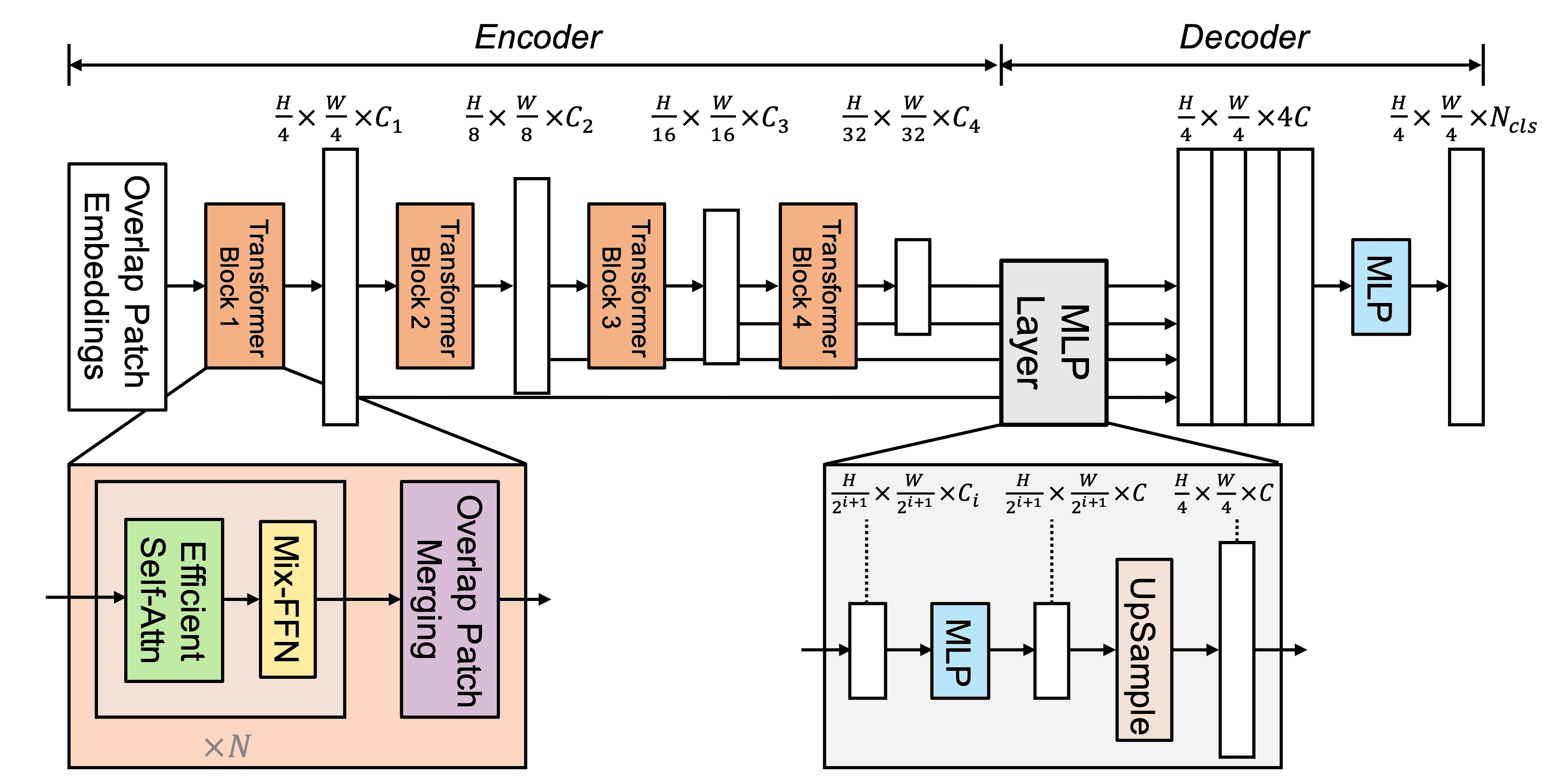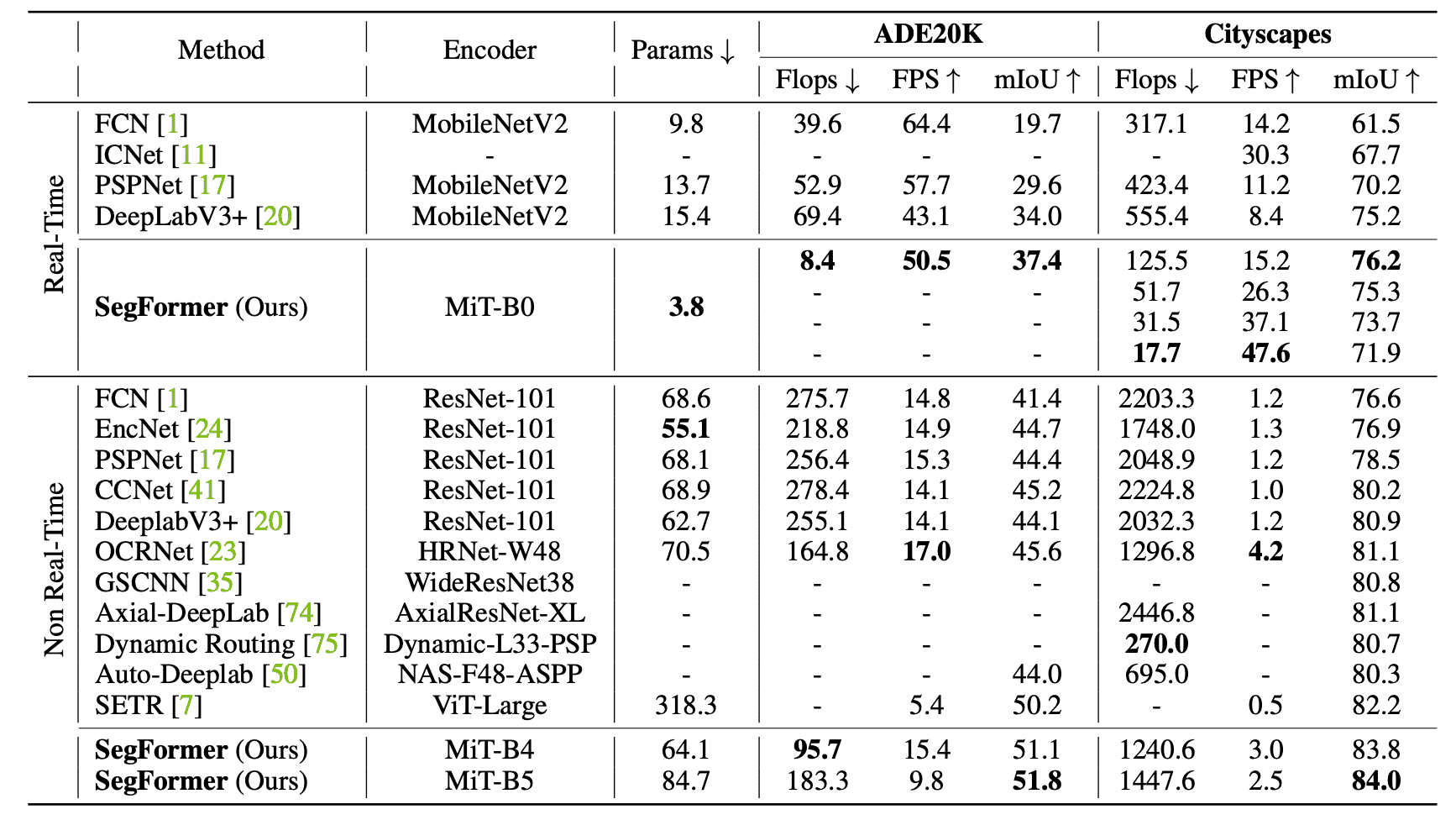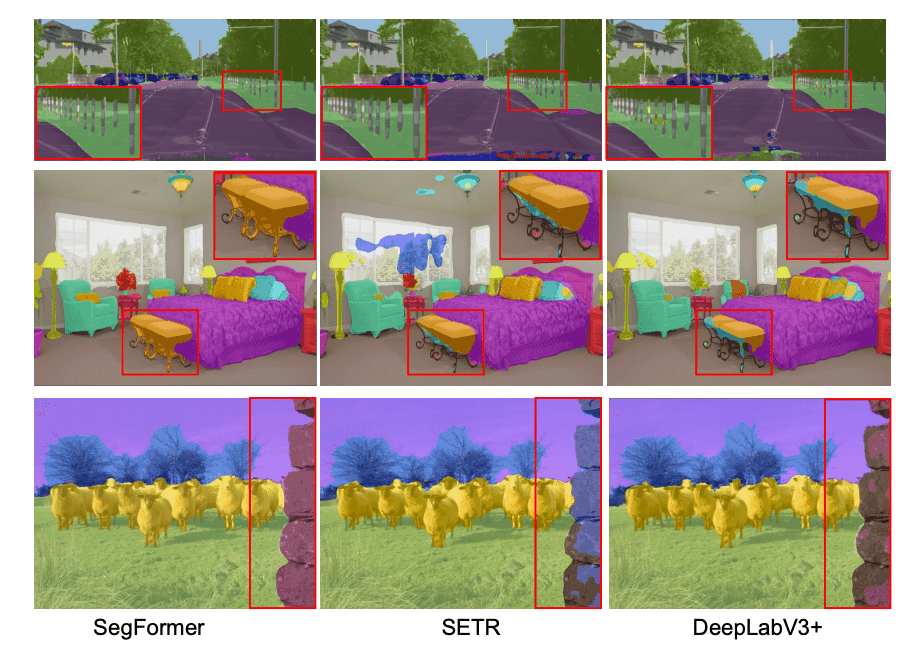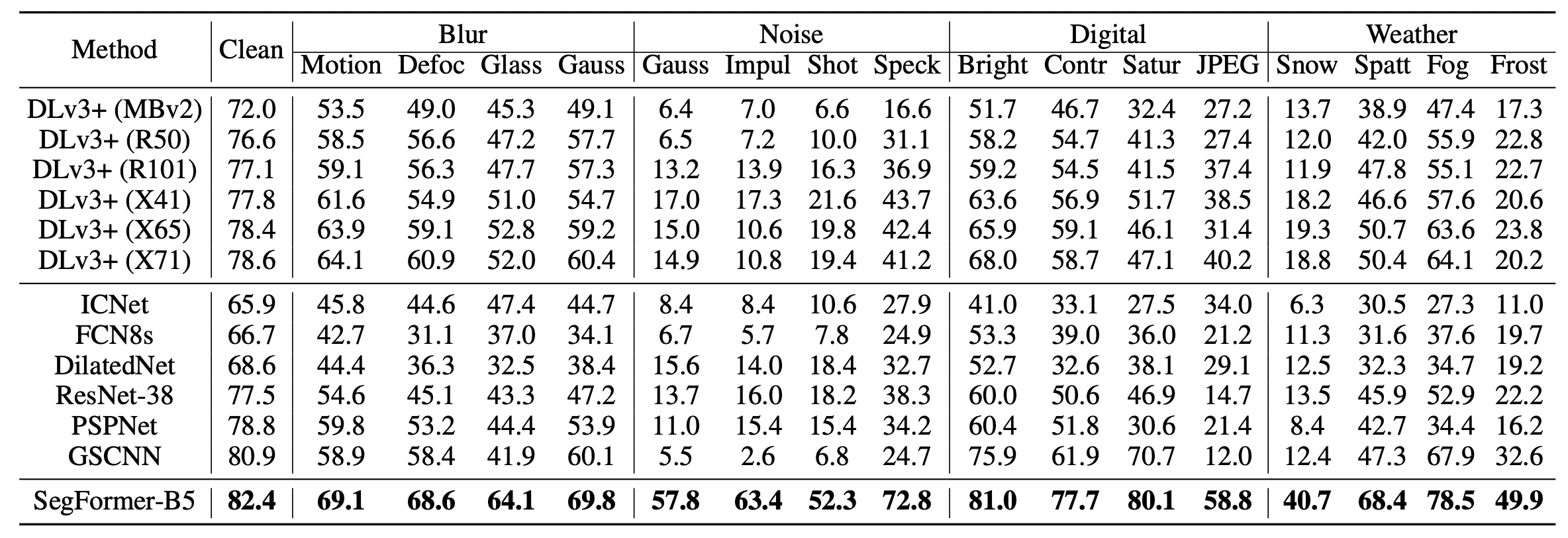# Catch up on the latest AI articles# SegFormer: Segmentation With Transformer

3 main points
✔️ We developed a Transformer-based segmentation model, SegFormer.
✔️ The hierarchical Transformer is used for the encoder to output multi-scale features, and the decoder is a simple MLP that combines each output to output advanced representation quantities.
✔️ SegFormer recorded SOTA despite its low computational cost compared to conventional methods.

written by Enze XieWenhai WangZhiding YuAnima AnandkumarJose M. AlvarezPing Luo
(Submitted on 31 May 2021 (v1), last revised 28 Oct 2021 (this version, v3))

Subjects: Computer Vision and Pattern Recognition (cs.CV); Machine Learning (cs.LG)code：The images used in this article are from the paper, the introductory slides, or were created based on them.

## background

Semantic segmentation is one of the major research areas in computer vision and has various applications. Unlike normal image classification, it categorizes images at the pixel level. Currently, the main model is based on FCN (Fully Connected Network) and various derivations exist. Also, due to its affinity with image classification, better backbone architectures have been studied as image classification progresses. On the other hand, the recent success of Transformer in the field of natural language processing has led to attempts to apply Transformer to image recognition as well. Therefore, in this paper, we developed SegFormer, a Transformer-based segmentation model that considers efficiency, accuracy, and robustness.

## technique

SegFormer consists of (1) a hierarchical Transformer encoder that generates high-resolution and low-resolution features and (2) a lightweight MLP decoder that combines those multi-scale features to generate a segmentation mask as shown in the figure below. Given an image of $H \times W \times 3$, we split it into $4 \times 4$ patches. These are then used as input to the encoder, which outputs features of {$1/4, 1/8, 1/16, 1/32$} scale of the original image size. These features are put into the decoder, which finally predicts a segmentation mask of $\frac{H}{4} \times \frac{W}{4} \times N_{cls}$. However, $N_{cls}$ is the number of categories.### Hierarchical Transformer encoder

In this paper, we have designed a series of Mix Transformer encoders (MiT), MiT-B0 to MiT-B5. They have the same architecture but different sizes, with MiT-B0 being the lightest and fastest to guess, and MiT-B5 being the largest and best performing.

### hierarchical feature representation

Unlike Vision Transformer (ViT), which generates a single resolution feature map, this module aims to generate multi-scale features like CNN. This is because high-resolution and low-resolution features generally improve the performance of semantic segmentation. More precisely, given an image $H \times W \times 3$, we perform patch merging to generate $\frac{H}{2^{i+1}} \times \frac{W}{2^{i+1}} \times C_i$ features $F_i$. However $i \in \{1,2,3,4\}, \ C_{i+1}>C_i$.

### overlap patch merging

Given a patch image, the patch merging process in ViT was to turn a patch of $N \times N \times 3$ into a vector of $1 \times 1 \ time C$. We mimicked this by transforming $F_1(\frac{H}{4} \times \frac{W}{4}{4} \times C_1)$ into $F_2(\frac{H}{8} \times \frac{W}{8} \times C_2)$. Since this process was designed to combine non-overlapping images, it does not preserve local continuity. Therefore, we performed overlap patch merging and adjusted the kernel size, stride, and padding size to produce features of the same size.

### Efficient Self-Attention

The bottleneck of the encoder calculation is the self-attention layer. In the traditional multi-head self-attention process, the attention is estimated as follows

$$Attention(Q,K,V)=Softmax(\frac{QK^T}{\sqrt{d_{head}}})V$$

However $Q,K,V$ are $N \times C$-dimensional vectors, $N=H \times W$. This computational complexity is $O(N^2)$, so it cannot be applied to large images. So, to reduce the sequence length, we introduce the following process.

$$\hat{K}=Reshape(\frac{N}{R}, C\cdot R)(K)$$

$$K=Linear(C\cdot R, C)(\hat{K})$$

where $K$ is the sequence to be reduced, $Reshape(\frac{N}{R}, C \cdot R)(K)$ transforms $K$ to size $\frac{N}{R}\times (C\cdot R)$, $Linear(C_{in}, C_{out})(\cdot)$ is the $C_{in }$-dimensional tensor to the $C_{out}$-dimensional tensor represents the linear layer outputting the $C_{out}$-dimensional tensor. The $R$ is the reduction rate, which reduces the computational complexity to $O(\frac{N^2}{R})$.

### Mix-FFN

ViT uses Positional Encoding (PE) to incorporate local information. However, since the resolution of PE is fixed, the performance degrades when the test data size is different. Therefore, we introduced Mix-FFN, which uses $3\times 3$ convolutional layers in a feed-forward network (FFN).

$$x_{out} = MLP(GELU(Conv_{3\times 3}(MLP(x_{in}))))+x_{in}$$

However, $x_{in}$ is the features from the self-attention module, and Mix-FFN combines each FFN with $3\times 3$ convolution layers and MLP.

### Lightweight All-MLP Decoder

Segformer employs a lightweight decoder consisting of only the MLP layer. This is possible because the hierarchical Transformer encoder has a wider effective receptive field (ERF) than a regular CNN.

$$\hat{F_i}=Linear(C_i,C)(F_i),\forall i$$

$$\hat{F_i}=Upsample(\frac{H}{4}\times \frac{W}{4})(\hat{F_i}),\forall i$$

$$F=Linear(4C,C)(Concat(\hat{F_i})),\forall i$$

$$M=Linear(C,N_{cls})(F)$$

However, $M$ is a prediction mask.

## experiment

Public data Cityscapes, ADE20K and COCO-Stuff were used for the experiments. Mean IoU (mIoU) was adopted as the evaluation index.

The performance comparison between ADE20K and the existing SOTA model in Cityscapes is shown in the table below. The upper side is Real-Time mode with a lighter structure for computation speed and the lower side is Non-Real-Time mode for performance.

For both datasets, the performance is high despite the small number of parameters compared to the previous model, which improves both computational speed and accuracy.The qualitative results are shown in the figure below: SegFormer's encoder can incorporate more precise features, storing more detailed texture information, and the guess results can predict object boundaries and other details more precisely.Finally, robustness is important for automated driving and other applications. To evaluate this, we experimented on the Cityscapes-C dataset, where we added various noises to the Cityscapes validation data. The results are shown in the table below.

The SegFormer shows a significantly higher IoU than previous models, and the results are promising for applications in safety-critical industries.## summary

In this paper, we proposed SegFormer, a segmentation model using Transformer. Compared with the existing models, SegFormer could obtain high performance with high efficiency by eliminating the heavy computation processing part. We also found that it has high robustness. Although the number of parameters has been significantly reduced, it is unclear whether it works on edge devices with small memory capacity, and determining this is a future challenge.

If you have any suggestions for improvement of the content of the article,